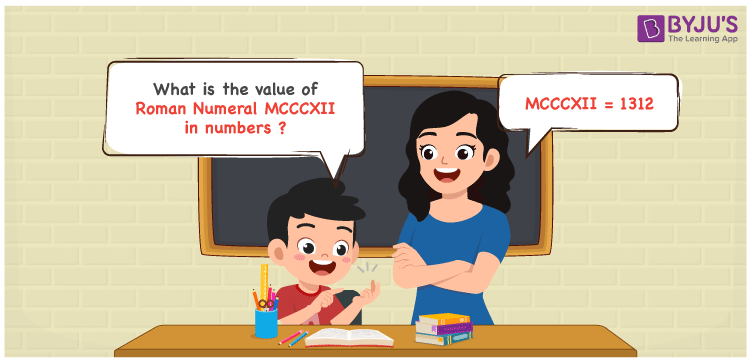Checkout JEE MAINS 2022 Question Paper Analysis : Checkout JEE MAINS 2022 Question Paper Analysis :

# MCCCXII Roman Numerals

MCCCXII Roman Numerals is 1312. Roman Numerals are the letters used by the ancient Romans to denote numbers. Students are recommended to download Roman Numerals Conversion in PDF format for quick and easy conversion of Roman Numerals into numbers. This also helps them to obtain in-depth knowledge on representing the Roman letters in a descriptive manner. Let us learn how to write the numerical value of MCCCXII Roman Numerals here.

 Number Roman Numeral 1312 MCCCXII

## How to Write MCCCXII Roman Numerals?The following methods can be used to express the numerical value of MCCCXII Roman Numerals

First Method:

• Break the Roman Numerals into single letters
• MCCCXII = M + C + C + C + X + I + I
• Write the numerical value of each letter and add/subtract them
• MCCCXII = 1000 + 100 + 100 + 100 + 10 + 1 + 1 = 1312

Second Method:

In this method, we consider the groups of Roman Numerals for addition or subtraction

• MCCCXII = M + CCC + X + II = 1000 + 300 + 10 + 2 = 1312
• Hence the numerical value of MCCCXII Roman Numerals is 1312

## Video Lesson on Roman Numerals## Related Articles

Roman Numerals

Roman Numerals 1 to 50

Roman Numerals 1 to 100

Roman Numerals 1 to 1000

L Roman Numeral

Roman Numeral XXXIX

## Frequently Asked Questions on MCCCXII Roman Numerals

### What is MCCCXII Roman Numerals?

MCCCXII Roman Numerals is 1312.

### What is the sum of 1000 + 312 in Roman Numerals?

1000 + 312 = 1312. The number 1312 in Roman Numerals is MCCCXII. Hence the sum of 1000 + 312 in Roman Numerals is MCCCXII.

### Does the number 1312 represent the Roman Numeral MCCCXII?

Yes. The number 1312 represents the Roman Numeral MCCCXII.